# The Harmonic Series

Previous: Introduction: Telescoping and Harmonic Series

Next: The Telescoping Series

Another useful example of a series whose convergence we can determine based on the behaviour of the partial sums is the harmonic series, whose infinite sum is given by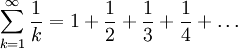Do you think this infinite series converges? The terms of the sequence are monotonically decreasing, so one might guess that the partial sums would in fact converge to some finite value and hence the sequence would converge.

### Plotting the Partial Sums of the Harmonic Series

The widget below plots the partial sums of the harmonic series for a chosen n. That is, it plots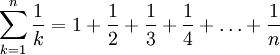for a value of n that you provide. Does it look like the partials sums are converging?

### Convergence of the Harmonic Series

There are a few different ways to to determine whether the harmonic series converges, but we will investigate this question using the definition of convergence above. Let's look at a few of the partial sums, and see if we can find a pattern. For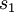andwe have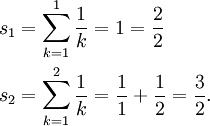Moving ahead to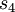we find (using highlighting to bring attention to what changes are made to create our inequality)We are beginning to see a pattern now. If we work ahead to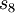, we obtainHence, it can be shown that in general,Astends to infinity, the partial sums go to infinity. Hence, using the definition of convergence of an infinite series, the harmonic series is divergent.

Alternate proofs of this result can be found in most introductory calculus textbooks, which the reader may find helpful. In any case, it is the result that students will be tested on, not its derivation.

Previous: Introduction: Telescoping and Harmonic Series

Next: The Telescoping Series